Pruning Filters for Efficient Convnets

Compressing deep neural nets

1.实现原始网络，并将其训练到收敛，保存权重

2.观察对每一层的权重，判断其对模型的贡献大小，删除贡献较小的kernel，评判标准可以是std，sum(abs)，mean等

3.当删除部分kernel后，会导致输出层的channel数变化，需要删除输出层对应kernel的对应channel

4.构建剪枝后的网络，加载剪枝后的权重，与原模型对比精准度。

5.使用较小的学习率，rebirth剪枝后的模型

6.重复第1步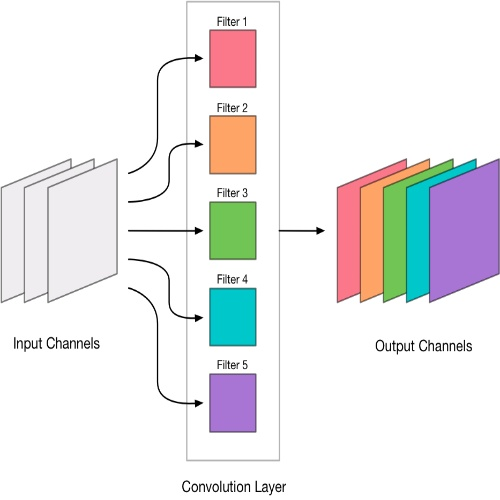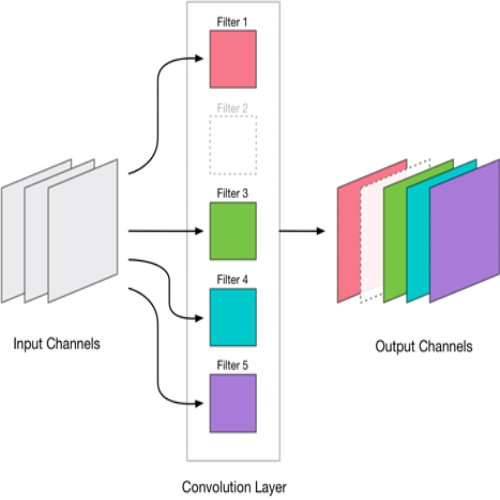conv层在接续全连接层前，会先reshape为一个维度。假设conv层输出为 (h,w,c)，其会reshape为 h*w*c， 假设删除的kernel下标为[ 2，5，7]，对应的conv输出通道也会减少 [2，5，7] 。reshape后 会减少 [2，5，7，...，h*w*2，h*w*5，h*w*7]。

### 一个使用mnist的简单示例

``````# 读取保存的权重和所有训练的var
model_path = './checkpoints/net_2018-12-19-10-05-17.ckpt-99900'
``````{'conv1/biases': ,
'conv1/weights': [3, 3, 1, 16],
'conv2/biases': ,
'conv2/weights': [3, 3, 16, 32],
'conv3/biases': ,
'conv3/weights': [3, 3, 32, 32],
'fc1/biases': ,
'fc1/weights': [512, 128],
'fc2/biases': ,
'fc2/weights': [128, 256],
'global_step': [],
'logits/biases': ,
'logits/weights': [256, 10]}``````
``````# 分析 conv1 的权重
# 计算每个kernel权重的和 (也可以使用其他指标，如std，mean等)
conv1_weight_sum = np.sum(conv1_weight, (0,1,2))
sort_conv1_weights = np.sort(conv1_weight_sum)
# 绘制conv1的
x = np.arange(0,len(sort_conv1_weights),step=1)
plt.plot(x,sort_conv1_weights)
``````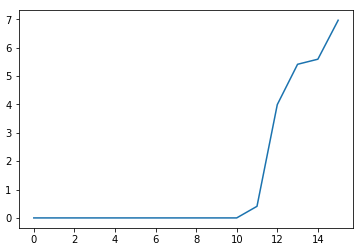``````# 保留权重和最大的8个kernel
pure_conv1_weight_index = np.where(conv1_weight_sum >= sort_conv1_weights)
pure_conv1_weight = conv1_weight[:,:,:,pure_conv1_weight_index]
# conv1对应的bias 也做相同处理
pure_conv1_bias = conv1_bias[pure_conv1_weight_index]``````
``````# 对后面接续的 conv 层的kernel做相同处理
conv2_weight = conv2_weight[:,:,pure_conv1_weight_index,:]``````

### 剪枝后结果对比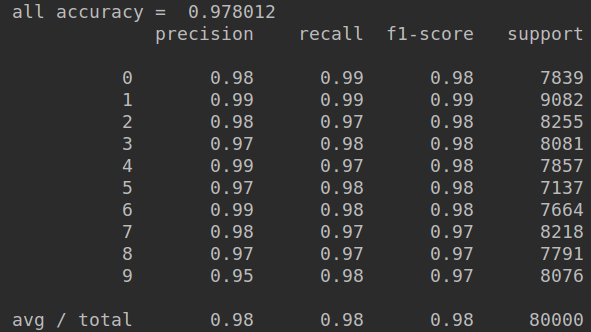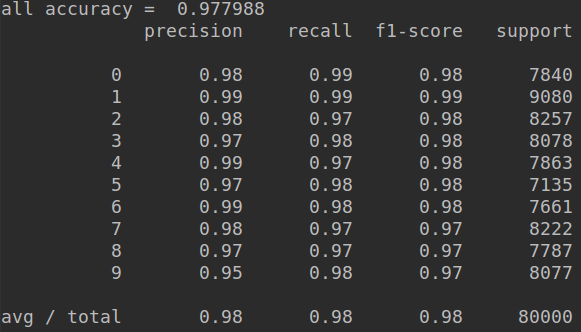jupyter文件及代码